Скачать презентацию Multi-Layer Channel Routing Complexity and Algorithm — Rajat

• Количество слайдов: 50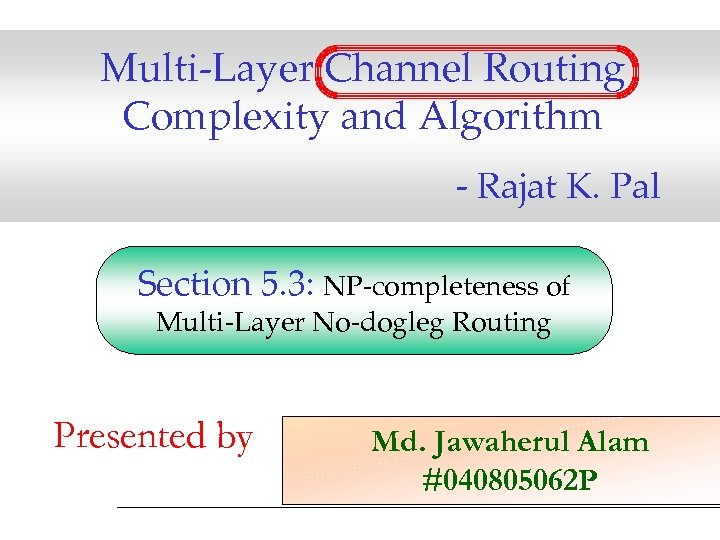Multi-Layer Channel Routing Complexity and Algorithm - Rajat K. Pal Section 5. 3: NP-completeness of Multi-Layer No-dogleg Routing Presented by Md. Jawaherul Alam #040805062 P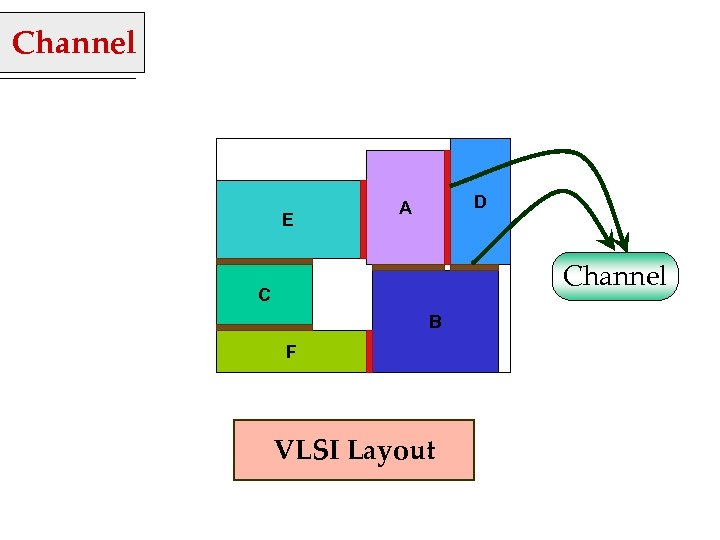Channel E D A Channel C B F VLSI Layout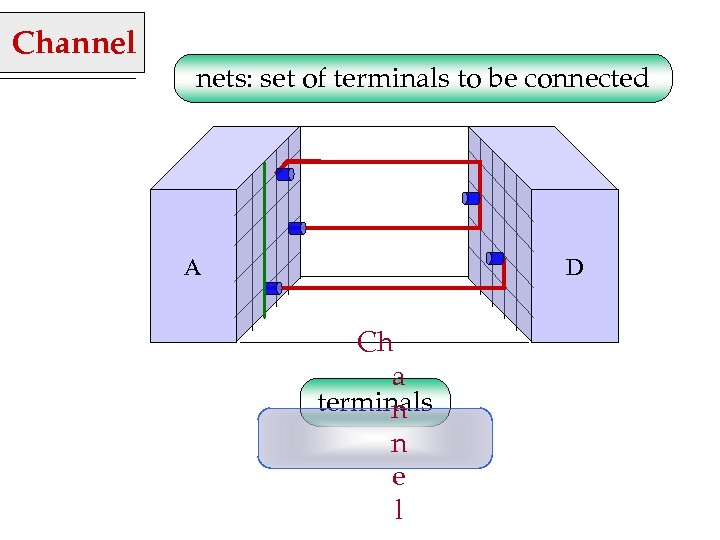Channel nets: set of terminals to be connected A D Ch a terminals n n e l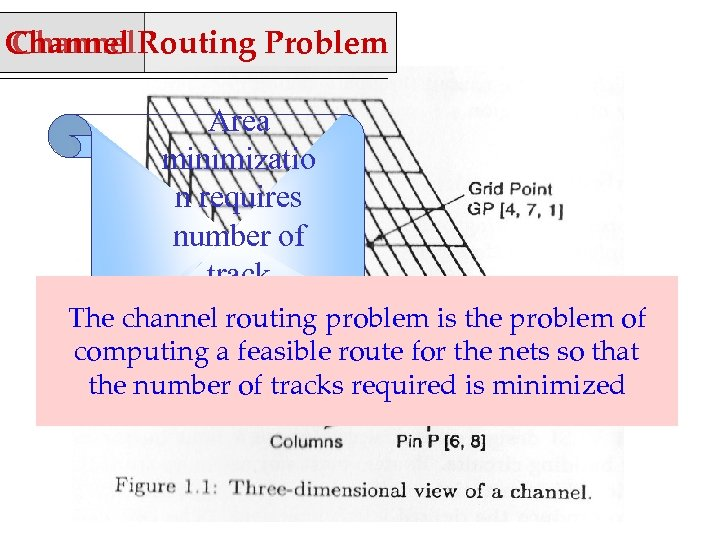Channel Routing Problem Channel Area minimizatio n requires number of track minimizatio The channel routing problem is the problem of computing an feasible route for the nets so that the number of tracks required is minimized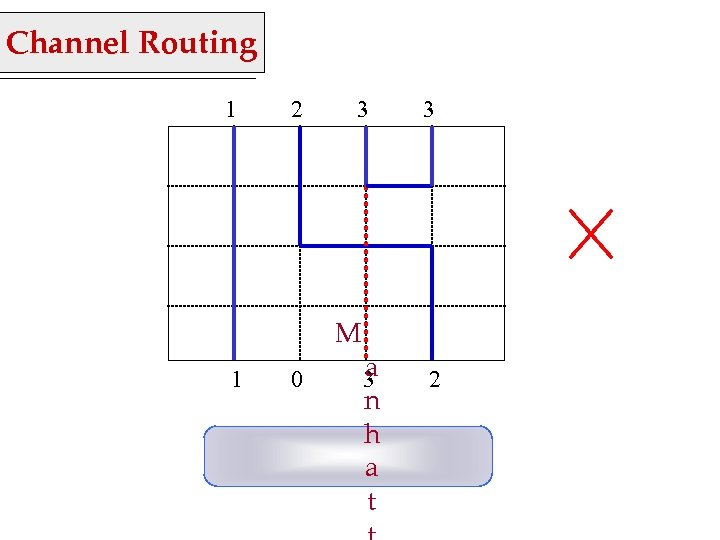Channel Routing 1 2 3 M 1 0 a 3 n h a t 3 2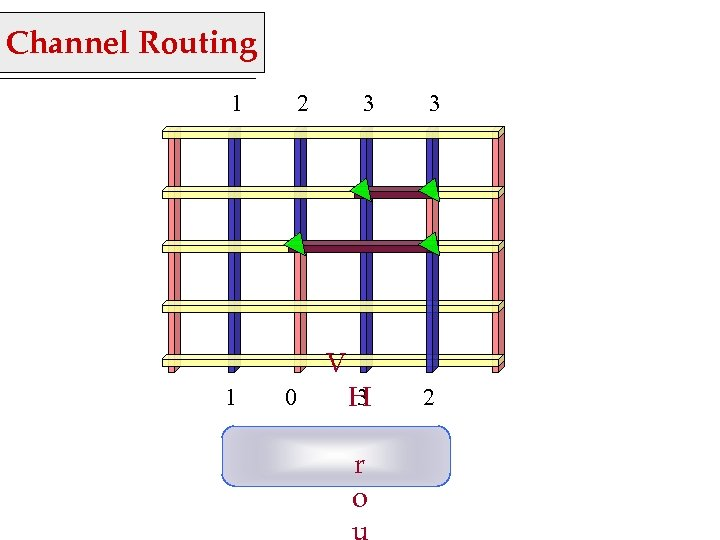Channel Routing 1 2 3 V 1 0 3 3 H 2 r o u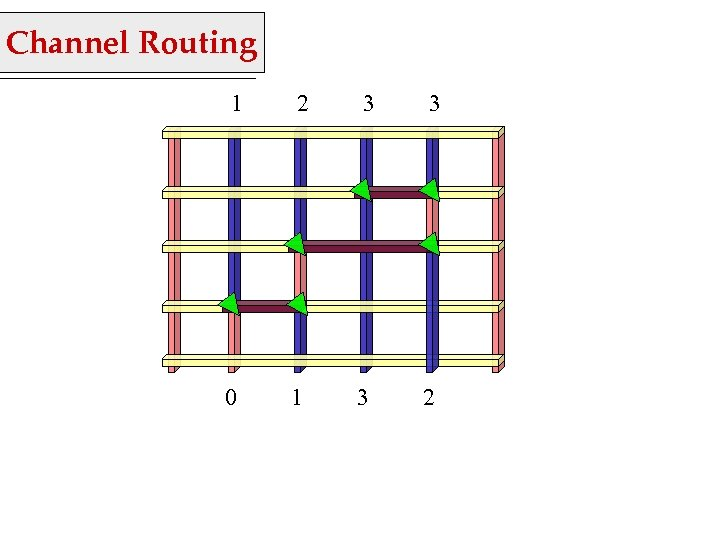Channel Routing 1 2 3 3 0 1 3 2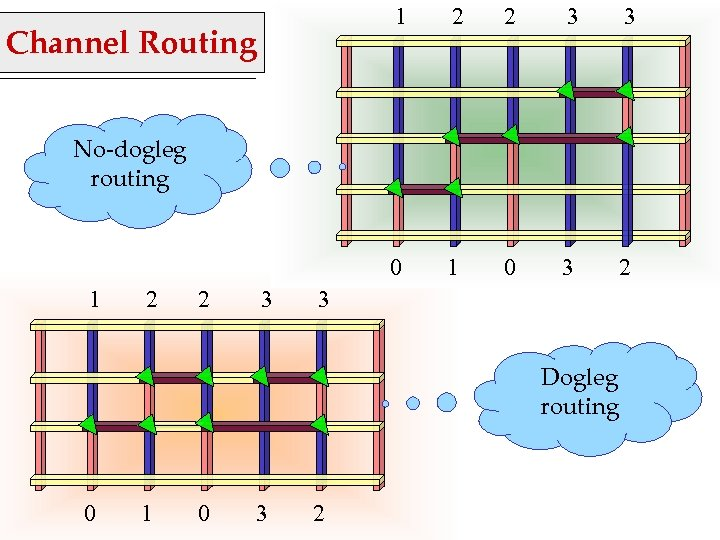1 2 3 3 0 Channel Routing 2 1 0 3 2 No-dogleg routing 1 2 2 3 3 Dogleg routing 0 1 0 3 2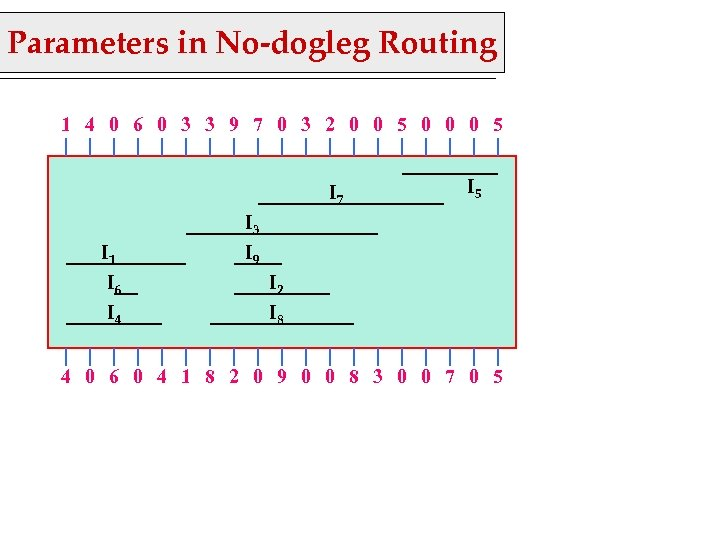Parameters in No-dogleg Routing 1 4 0 6 0 3 3 9 7 0 3 2 0 0 5 0 0 0 5 I 7 I 1 I 6 I 4 I 5 I 3 I 9 I 2 I 8 4 0 6 0 4 1 8 2 0 9 0 0 8 3 0 0 7 0 5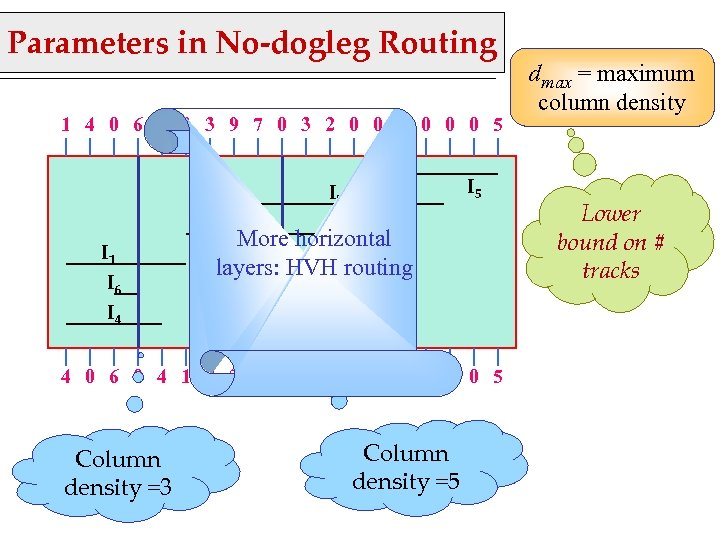Parameters in No-dogleg Routing 1 4 0 6 0 3 3 9 7 0 3 2 0 0 5 0 0 0 5 I 7 I 1 I 6 I 4 I 3 More horizontal I 9 layers: HVH routing I 2 I 8 4 0 6 0 4 1 8 2 0 9 0 0 8 3 0 0 7 0 5 Column density =3 Column density =5 dmax = maximum column density Lower bound on # tracks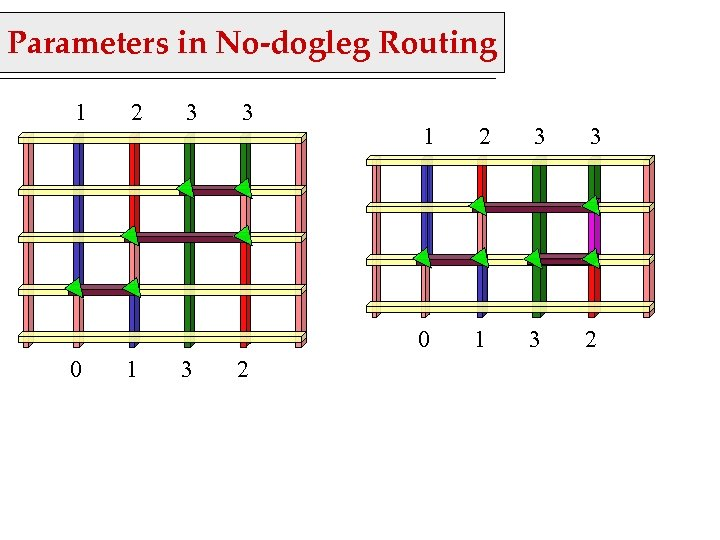Parameters in No-dogleg Routing 1 2 3 3 1 3 2 2 3 3 0 0 1 1 3 2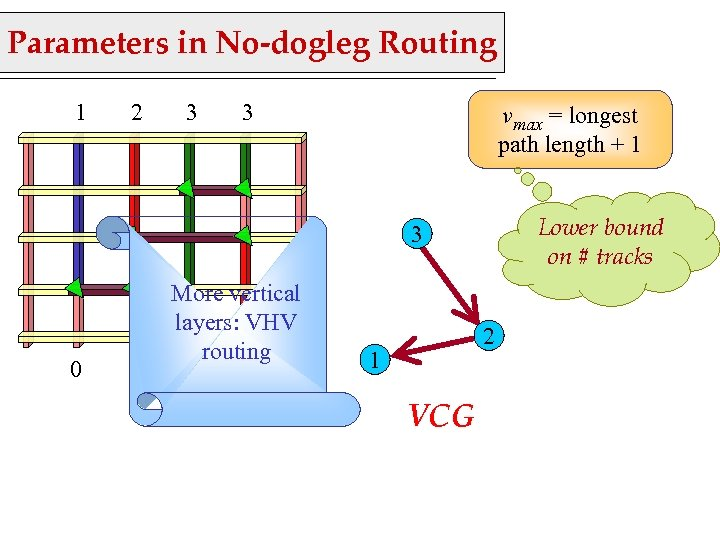Parameters in No-dogleg Routing 1 2 3 3 vmax = longest path length + 1 Lower bound on # tracks 3 0 1 More vertical layers: VHV routing 3 2 2 1 VCG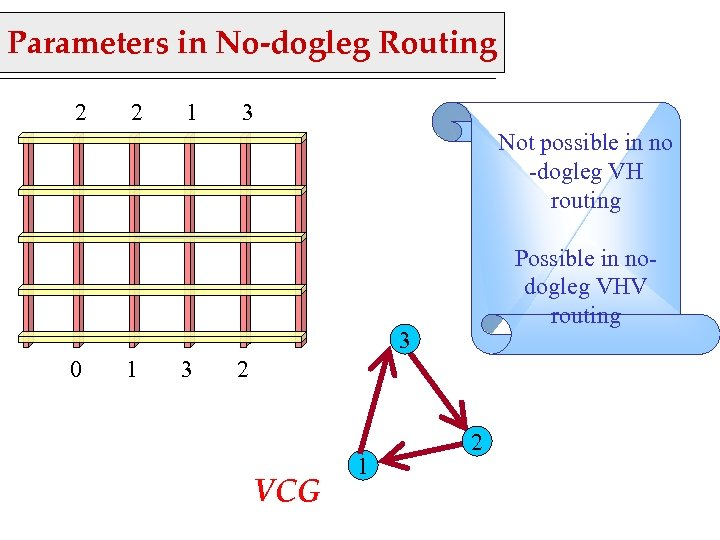Parameters in No-dogleg Routing 2 2 1 3 Not possible in no -dogleg VH routing Possible in nodogleg VHV routing 3 0 1 3 2 VCG 1 2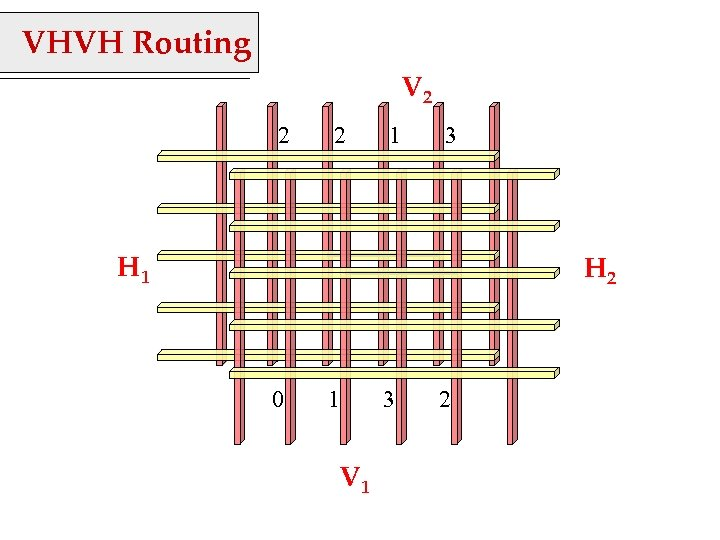VHVH Routing V 2 2 2 1 3 H 1 H 2 0 1 V 1 3 2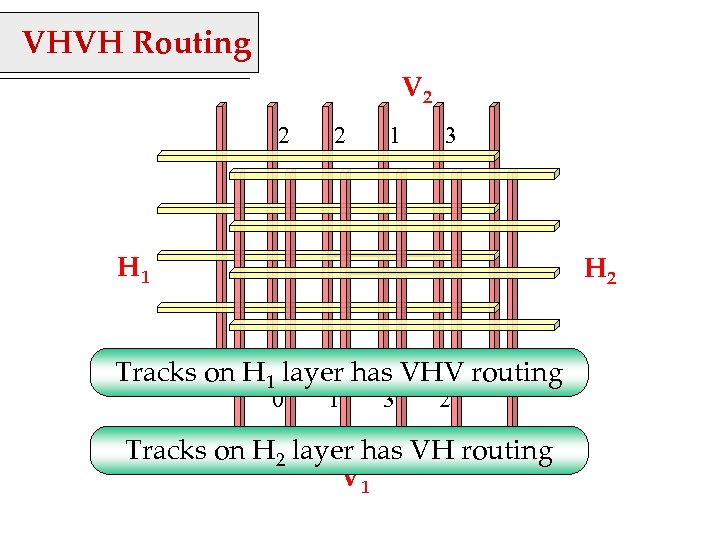VHVH Routing V 2 2 2 1 3 H 1 H 2 Tracks on H 1 layer has VHV routing 0 1 3 2 Tracks on H 2 layer has VH routing V 1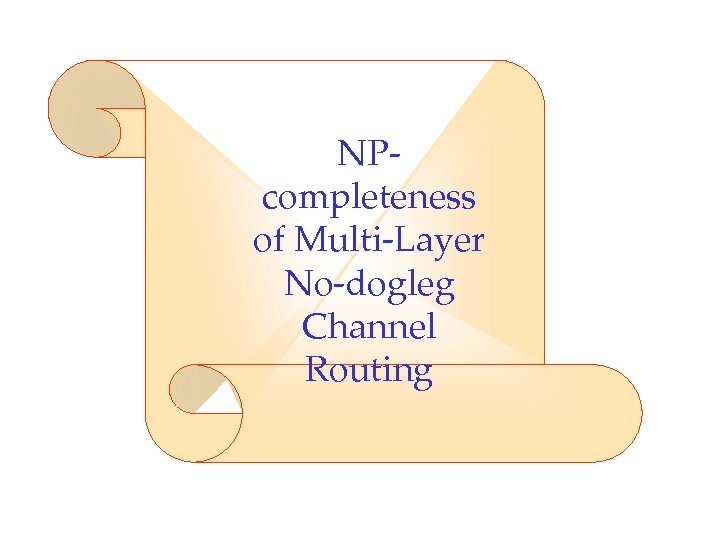NPcompleteness of Multi-Layer No-dogleg Channel Routing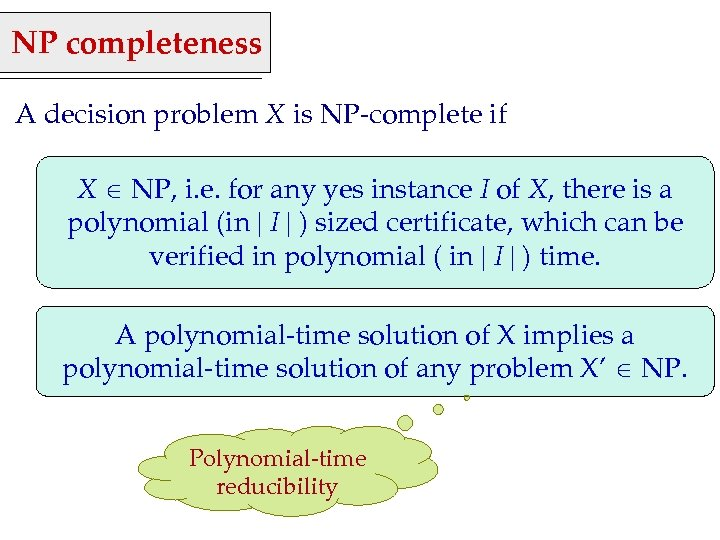NP completeness A decision problem X is NP-complete if X NP, i. e. for any yes instance I of X, there is a polynomial (in I ) sized certificate, which can be verified in polynomial ( in I ) time. A polynomial-time solution of X implies a polynomial-time solution of any problem X’ NP. Polynomial-time reducibility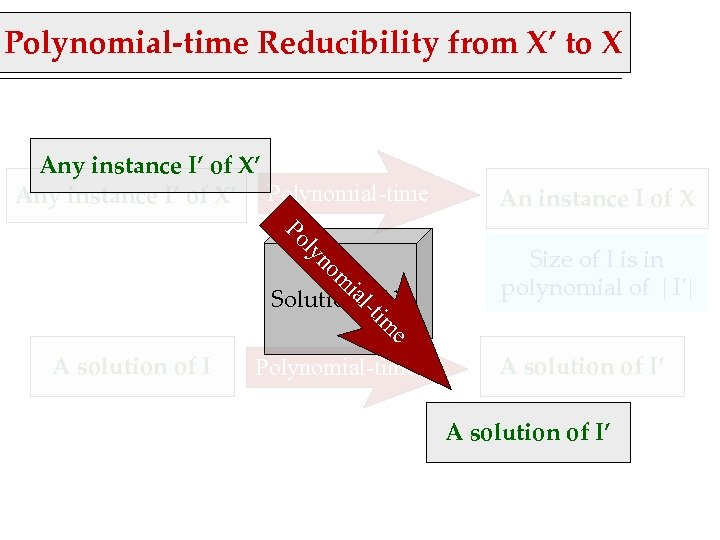Polynomial-time Reducibility from X’ to X Any instance I’ of X’ Polynomial-time om yn l Po Size of I is in polynomial of I’ e im l-t ia Solution of X An instance I of X A solution of I Polynomial-time A solution of I’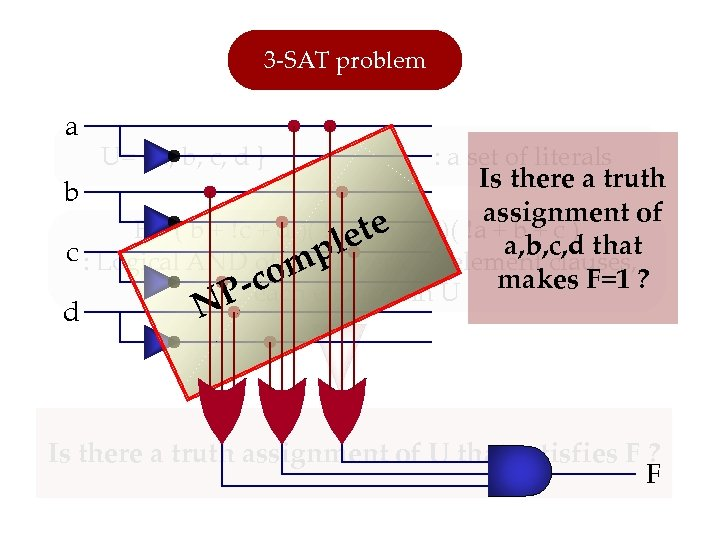3 -SAT problem a U= { a, b, c, d } : a set of literals Is there a truth b e + a )( aassignment of F = ( b + c + d )( d et b + +b+c) a, b, c, d that c : Logical AND of q number of 3 -element clauses, pl comelement in U makes F=1 ? P-each N d Is there a truth assignment of U that satisfies F ? F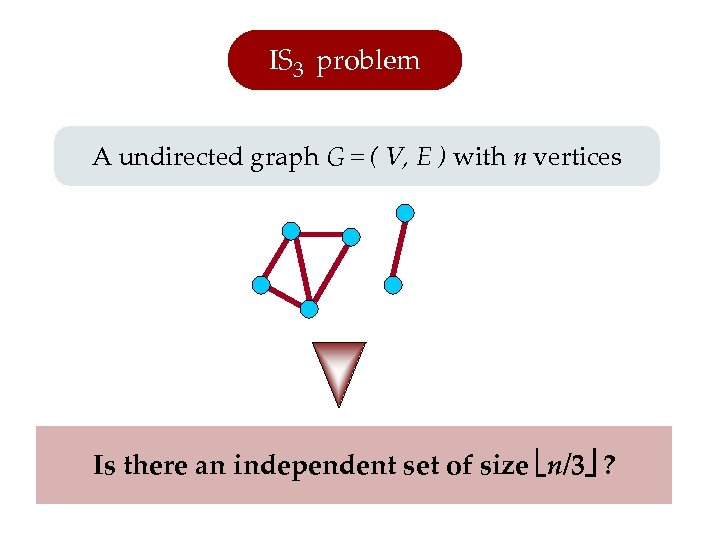IS 3 problem A undirected graph G = ( V, E ) with n vertices Is there an independent set of size n/3 ?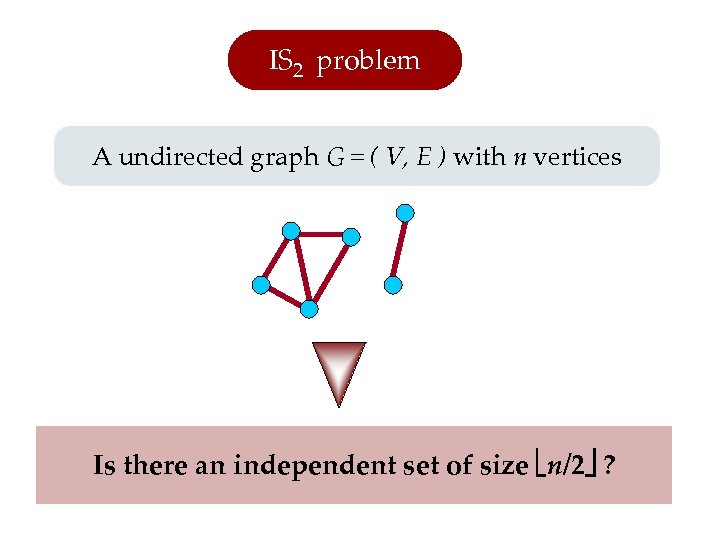IS 2 problem A undirected graph G = ( V, E ) with n vertices Is there an independent set of size n/2 ?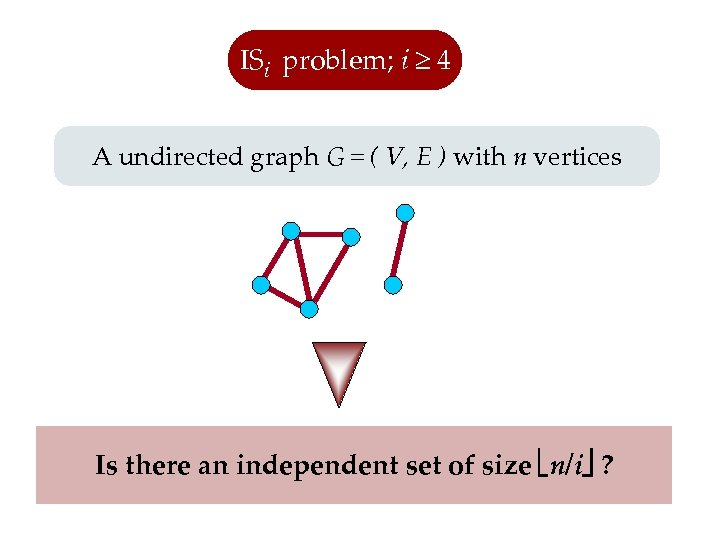ISi problem; i ≥ 4 A undirected graph G = ( V, E ) with n vertices Is there an independent set of size n/i ?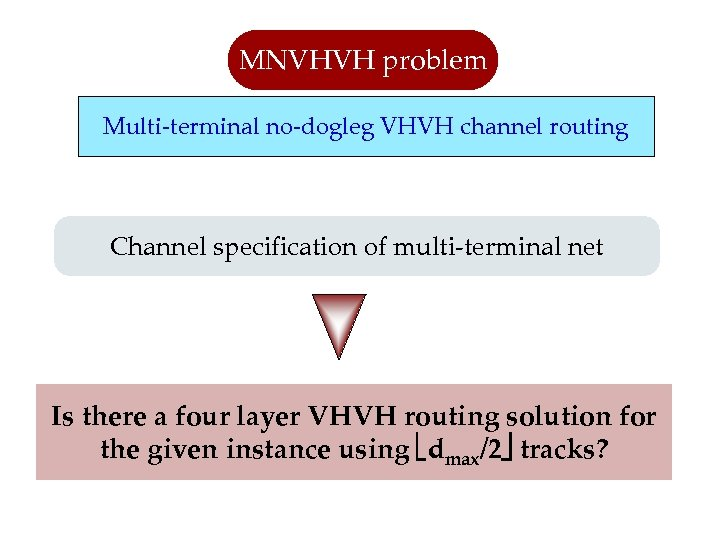MNVHVH problem Multi-terminal no-dogleg VHVH channel routing Channel specification of multi-terminal net Is there a four layer VHVH routing solution for the given instance using dmax/2 tracks?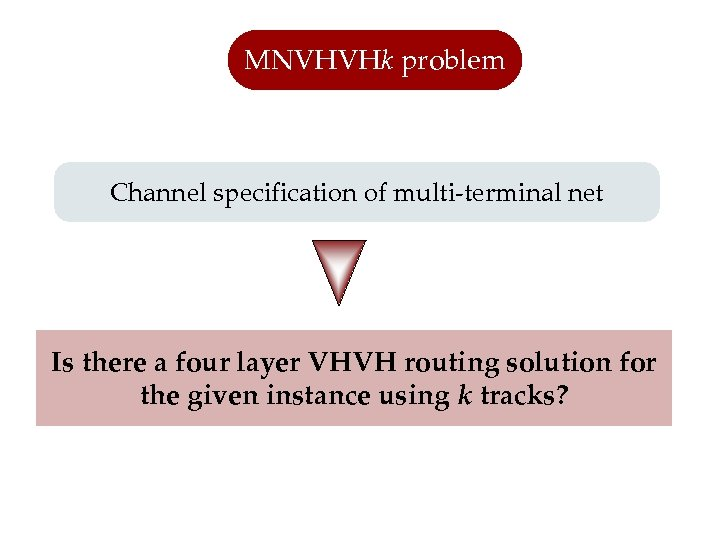MNVHVHk problem Channel specification of multi-terminal net Is there a four layer VHVH routing solution for the given instance using k tracks?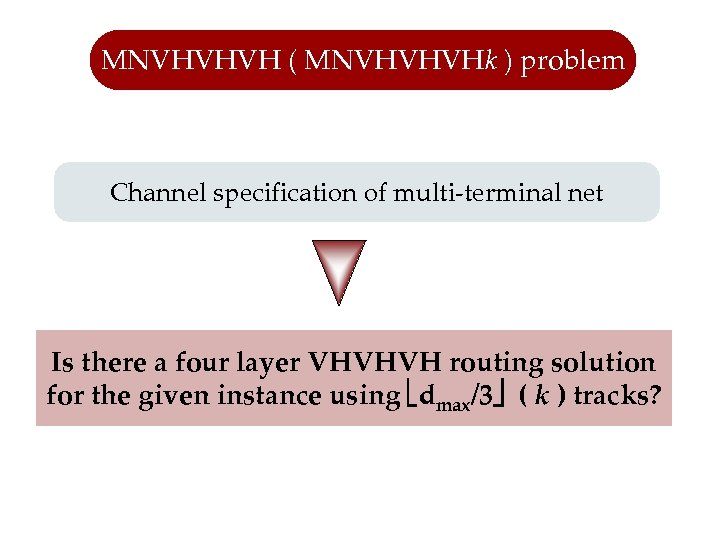MNVHVHVH ( MNVHVHVHk ) problem Channel specification of multi-terminal net Is there a four layer VHVHVH routing solution for the given instance using dmax/3 ( k ) tracks?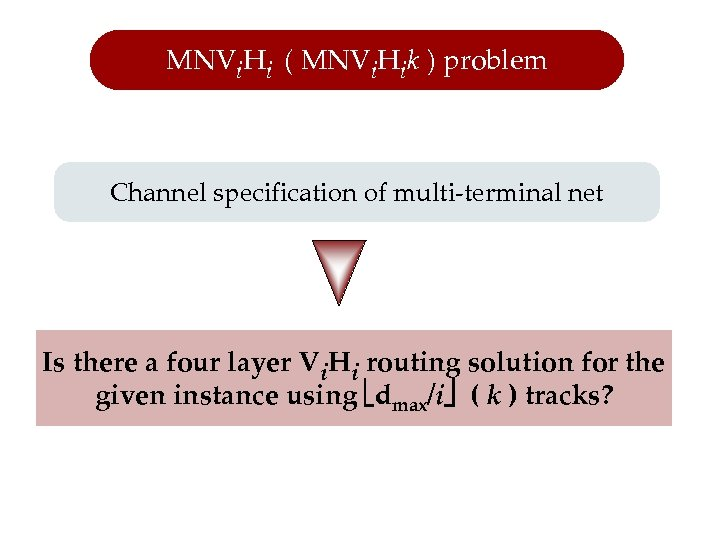MNVi. Hi ( MNVi. Hik ) problem Channel specification of multi-terminal net Is there a four layer Vi. Hi routing solution for the given instance using dmax/i ( k ) tracks?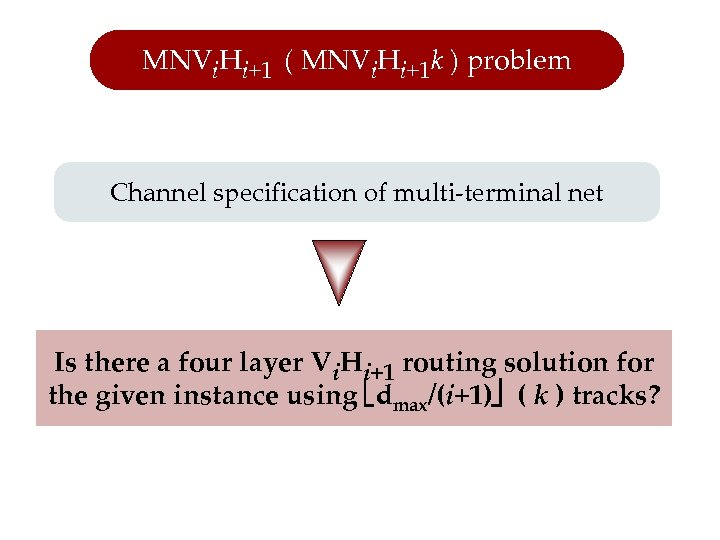MNVi. Hi+1 ( MNVi. Hi+1 k ) problem Channel specification of multi-terminal net Is there a four layer Vi. Hi+1 routing solution for the given instance using dmax/(i+1) ( k ) tracks?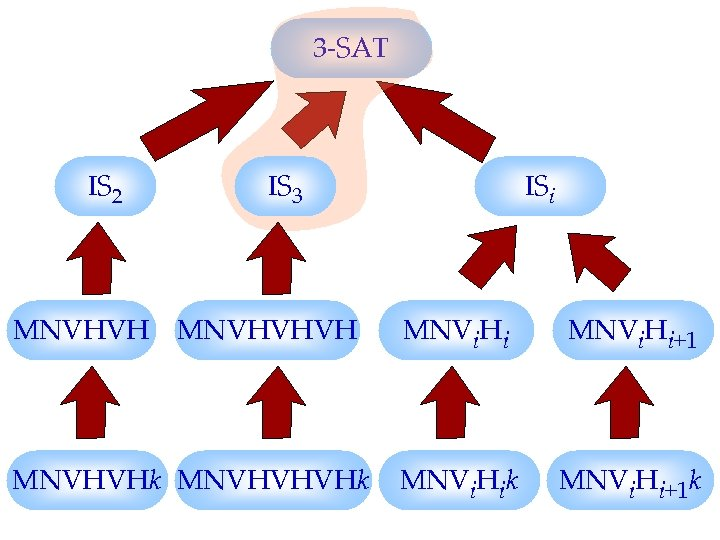3 -SAT IS 2 IS 3 ISi MNVHVHVH MNVi. Hi+1 MNVHVHk MNVHVHVHk MNVi. Hi+1 k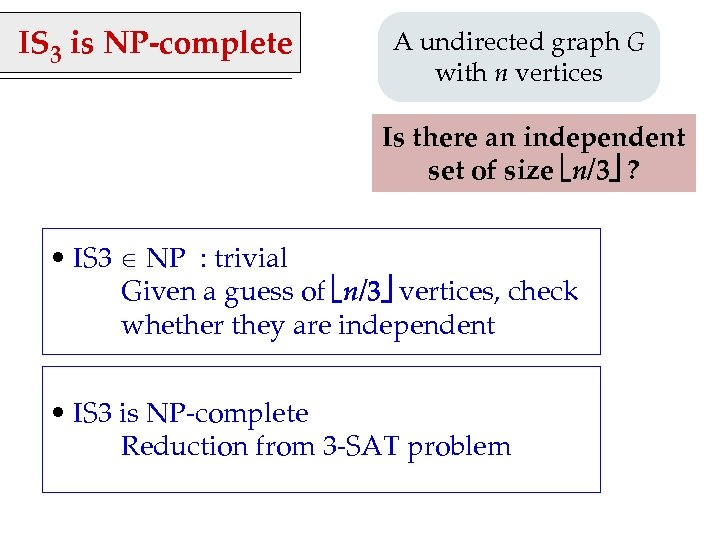IS 3 is NP-complete A undirected graph G with n vertices Is there an independent set of size n/3 ? • IS 3 NP : trivial Given a guess of n/3 vertices, check whether they are independent • IS 3 is NP-complete Reduction from 3 -SAT problem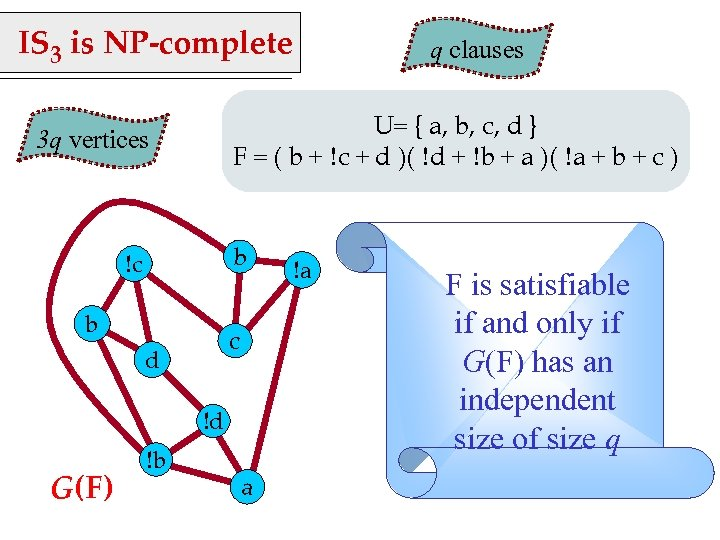IS 3 is NP-complete U= { a, b, c, d } F = ( b + c + d )( d + b + a )( a + b + c ) 3 q vertices b !c b c d !d G(F) !b q clauses a !a F is satisfiable if and only if G(F) has an independent size of size q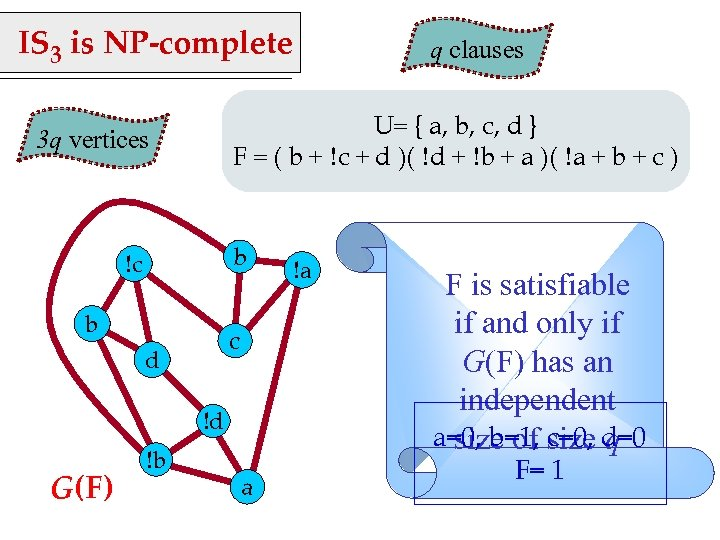IS 3 is NP-complete U= { a, b, c, d } F = ( b + c + d )( d + b + a )( a + b + c ) 3 q vertices b !c b c d !d G(F) !b q clauses a !a F is satisfiable if and only if G(F) has an independent a=0, b=1, size d=0 size of c=0, q F= 1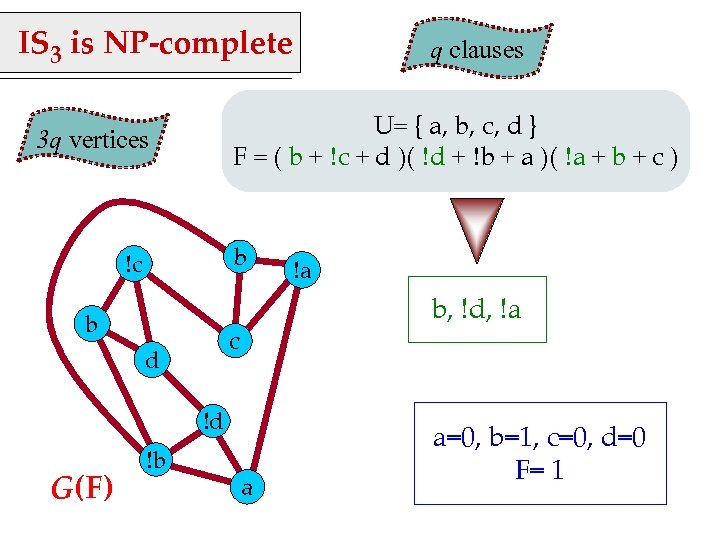IS 3 is NP-complete U= { a, b, c, d } F = ( b + c + d )( d + b + a )( a + b + c ) 3 q vertices b !c b !d G(F) !a b, !d, !a c d !b q clauses a a=0, b=1, c=0, d=0 F= 1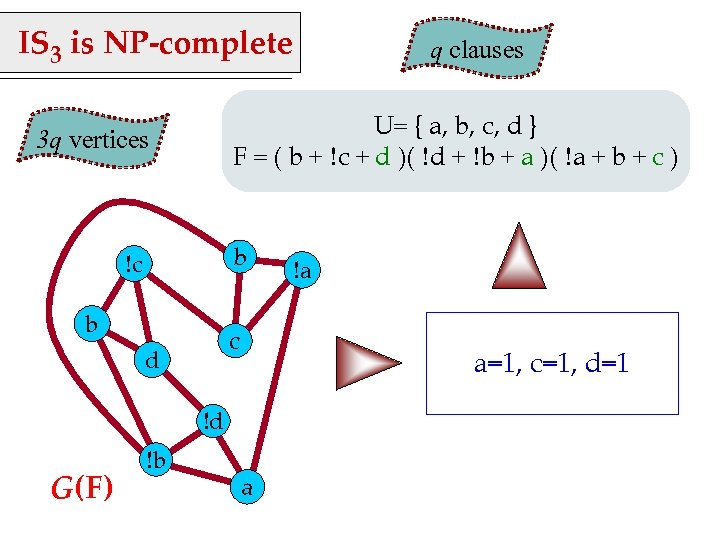IS 3 is NP-complete U= { a, b, c, d } F = ( b + c + d )( d + b + a )( a + b + c ) 3 q vertices b !c b c d G(F) !a a=1, c=1, d=1 !d !b q clauses a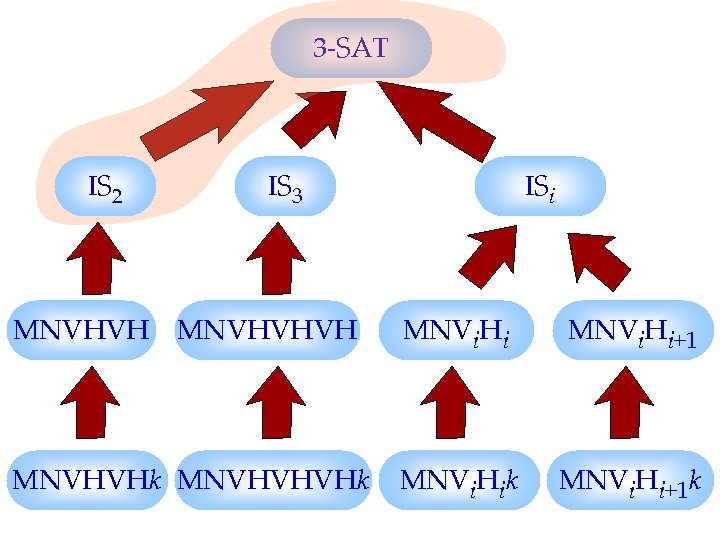3 -SAT IS 2 IS 3 ISi MNVHVHVH MNVi. Hi+1 MNVHVHk MNVHVHVHk MNVi. Hi+1 k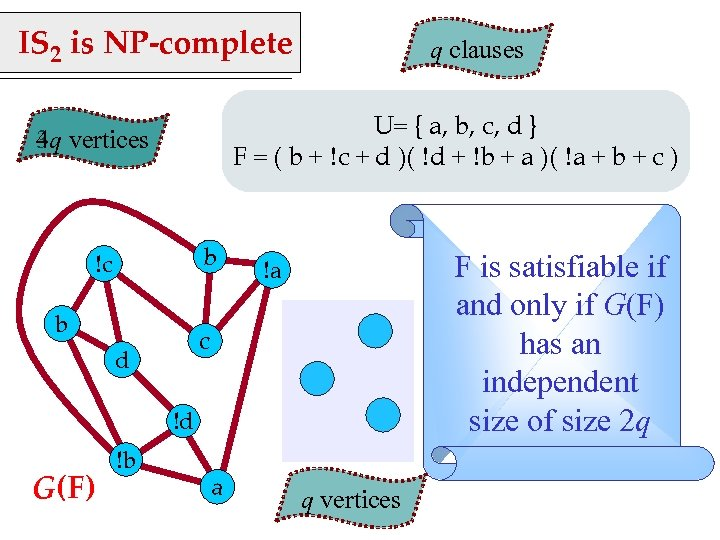IS 2 is NP-complete q clauses U= { a, b, c, d } F = ( b + c + d )( d + b + a )( a + b + c ) 4 q 3 q vertices b !c b F is satisfiable if and only if G(F) has an independent size of size 2 q !a c d !d G(F) !b a q vertices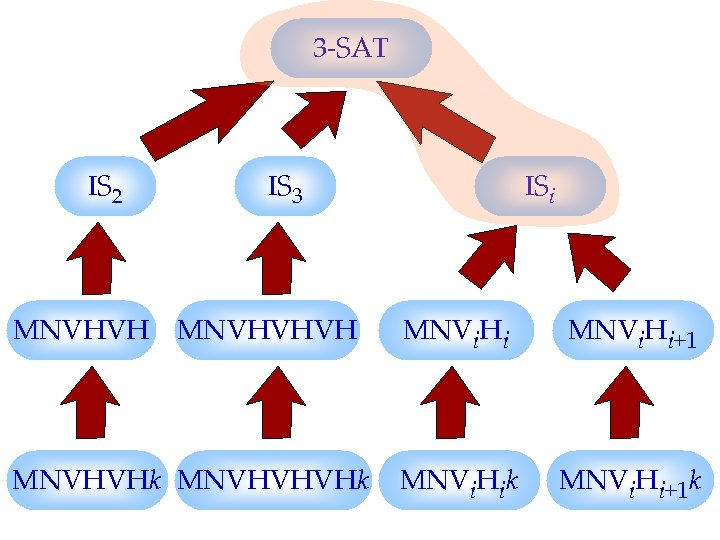3 -SAT IS 2 IS 3 ISi MNVHVHVH MNVi. Hi+1 MNVHVHk MNVHVHVHk MNVi. Hi+1 k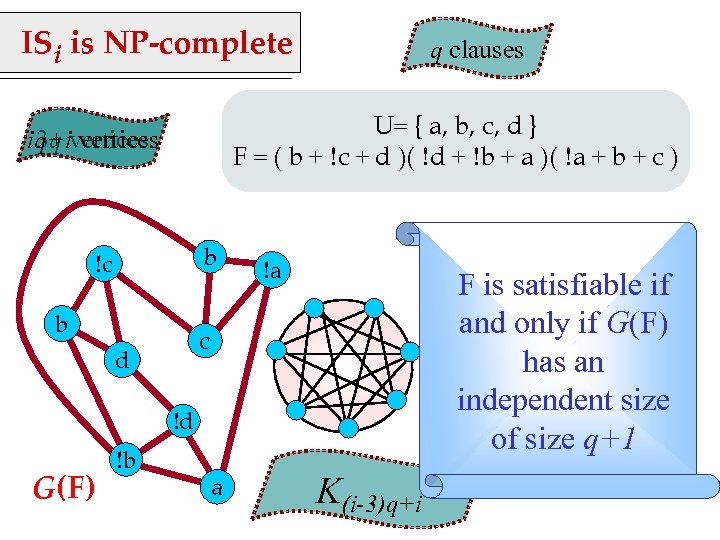ISi is NP-complete q clauses U= { a, b, c, d } F = ( b + c + d )( d + b + a )( a + b + c ) iq+ivertices 3 q vertices b !c b !a F is satisfiable if and only if G(F) has an independent size of size q+1 c d !d G(F) !b a K(i-3)q+i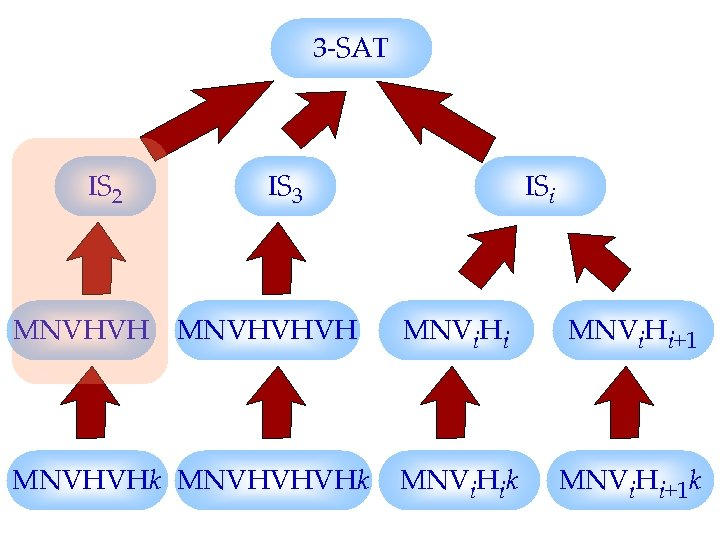3 -SAT IS 2 IS 3 ISi MNVHVHVH MNVi. Hi+1 MNVHVHk MNVHVHVHk MNVi. Hi+1 k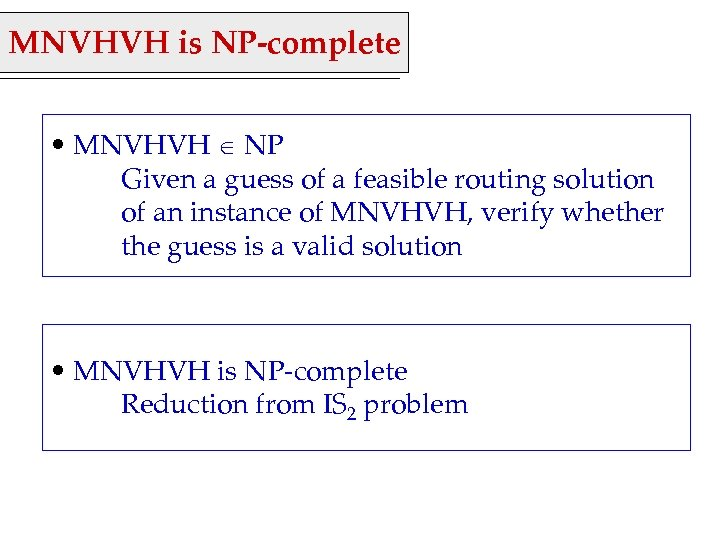MNVHVH is NP-complete • MNVHVH NP Given a guess of a feasible routing solution of an instance of MNVHVH, verify whether the guess is a valid solution • MNVHVH is NP-complete Reduction from IS 2 problem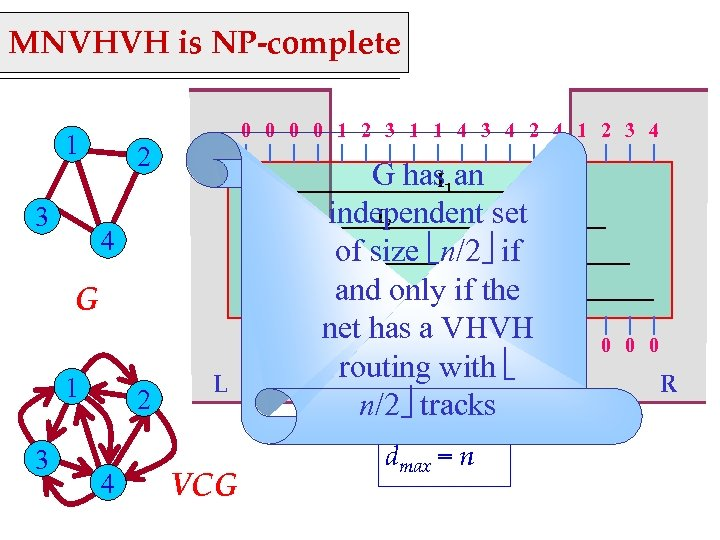MNVHVH is NP-complete 0 0 1 2 3 1 1 4 3 4 2 4 1 2 3 4 1 3 2 4 G 1 2 3 1 3 2 4 L VCG G has 1 an I I 2 independent set I 3 of size n/2 if I and only 4 if the net has a VHVH 4 2 1 1 3 4 1 4 3 4 routing. M with n/2 tracks dmax = n 2 0 0 R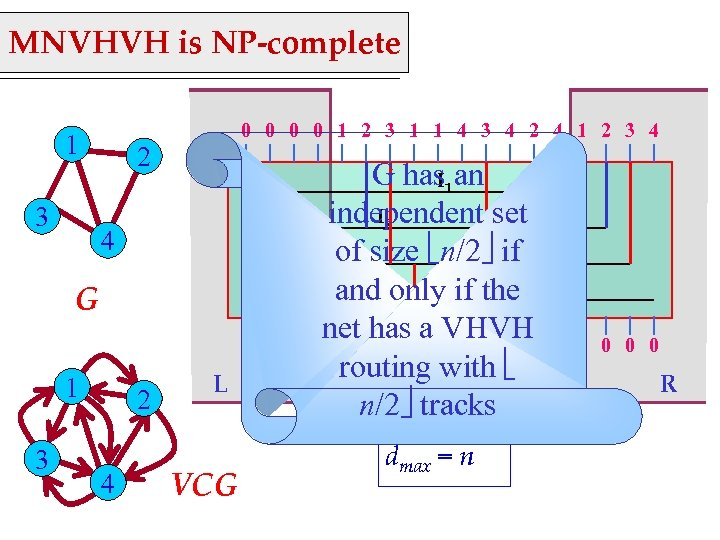MNVHVH is NP-complete 0 0 1 2 3 1 1 4 3 4 2 4 1 2 3 4 1 3 2 4 G 1 2 3 1 3 2 4 L VCG G has 1 an I I 2 independent set I 3 of size n/2 if I and only 4 if the net has a VHVH 4 2 1 1 3 4 1 4 3 4 routing. M with n/2 tracks dmax = n 2 0 0 R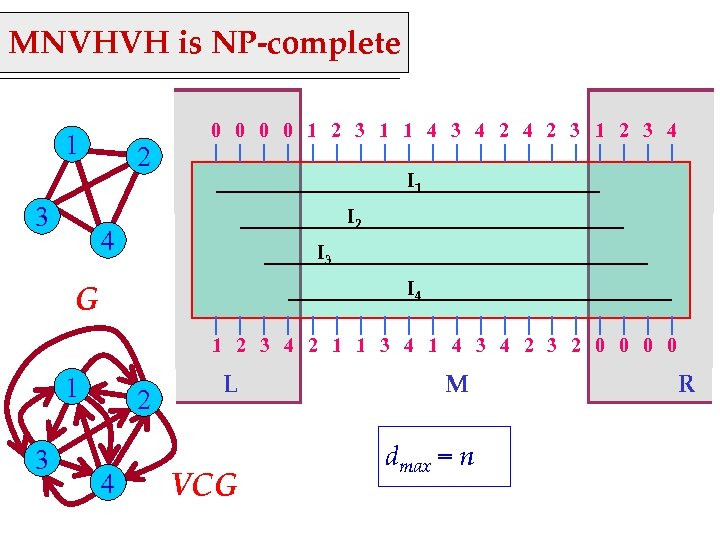MNVHVH is NP-complete 0 0 1 2 3 1 1 4 3 4 2 3 1 2 3 4 1 3 2 I 1 I 2 4 I 3 I 4 G 1 2 3 4 2 1 1 3 4 1 4 3 4 2 3 2 0 0 1 3 2 4 L VCG M dmax = n R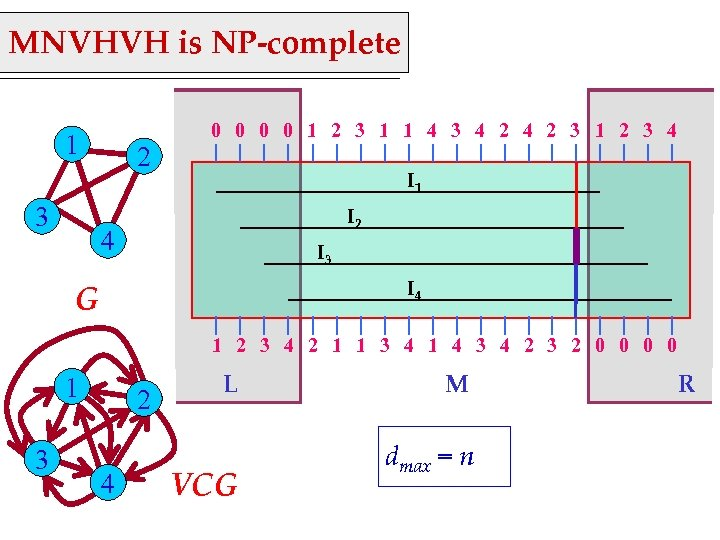MNVHVH is NP-complete 0 0 1 2 3 1 1 4 3 4 2 3 1 2 3 4 1 3 2 I 1 I 2 4 I 3 I 4 G 1 2 3 4 2 1 1 3 4 1 4 3 4 2 3 2 0 0 1 3 2 4 L VCG M dmax = n R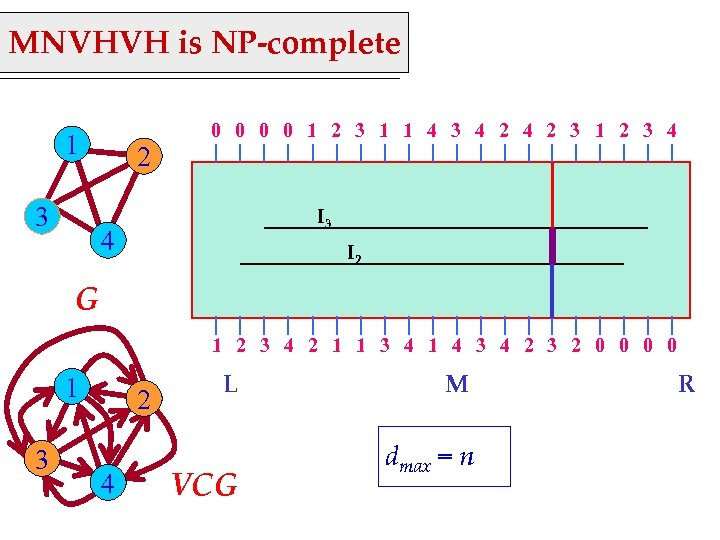MNVHVH is NP-complete 0 0 1 2 3 1 1 4 3 4 2 3 1 2 3 4 1 3 2 I 3 4 I 2 G 1 2 3 4 2 1 1 3 4 1 4 3 4 2 3 2 0 0 1 3 2 4 L VCG M dmax = n R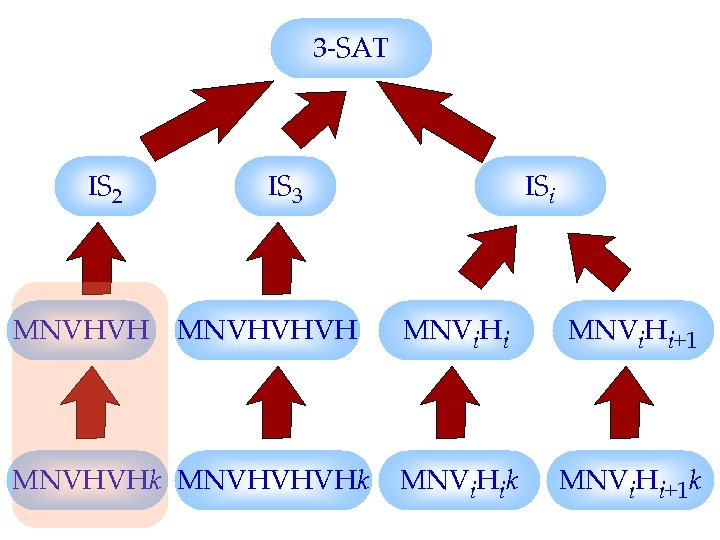3 -SAT IS 2 IS 3 ISi MNVHVHVH MNVi. Hi+1 MNVHVHk MNVHVHVHk MNVi. Hi+1 k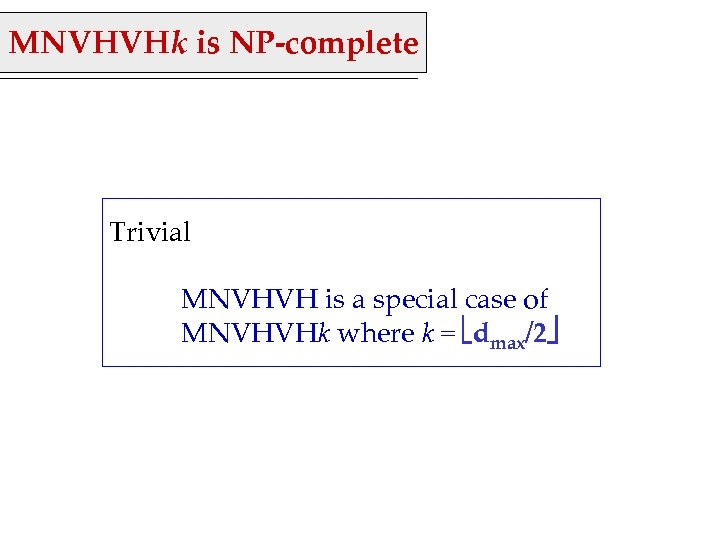MNVHVHk is NP-complete Trivial MNVHVH is a special case of MNVHVHk where k = dmax/2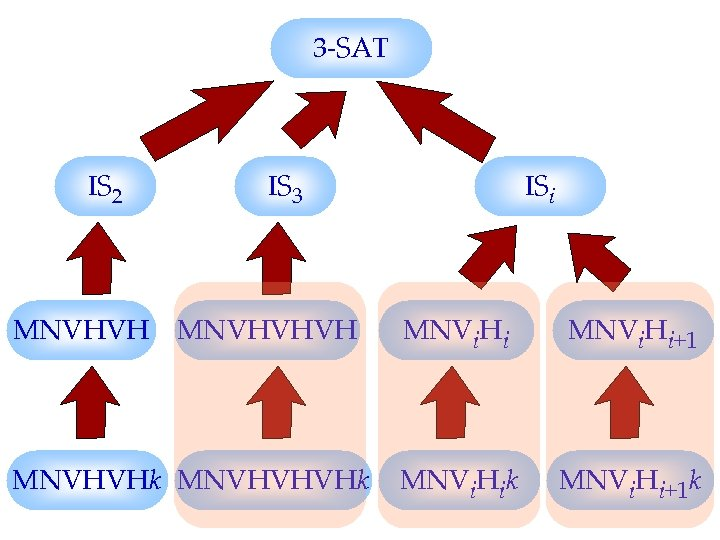3 -SAT IS 2 IS 3 ISi MNVHVHVH MNVi. Hi+1 MNVHVHk MNVHVHVHk MNVi. Hi+1 kThank You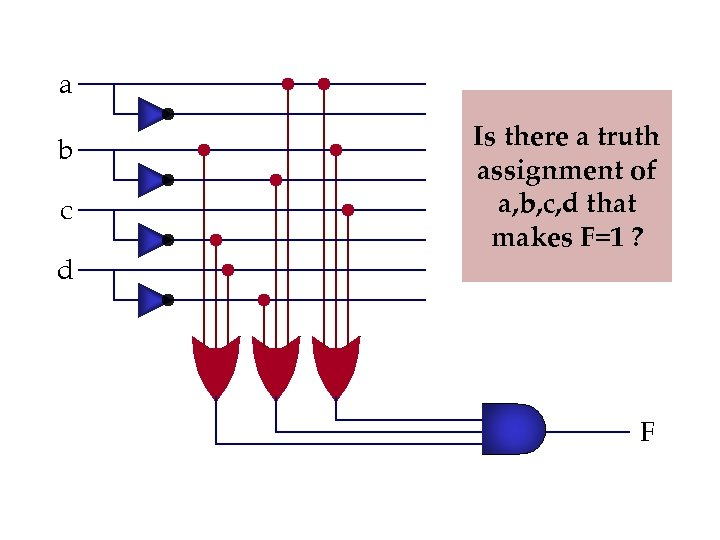a b c d Is there a truth assignment of a, b, c, d that makes F=1 ? F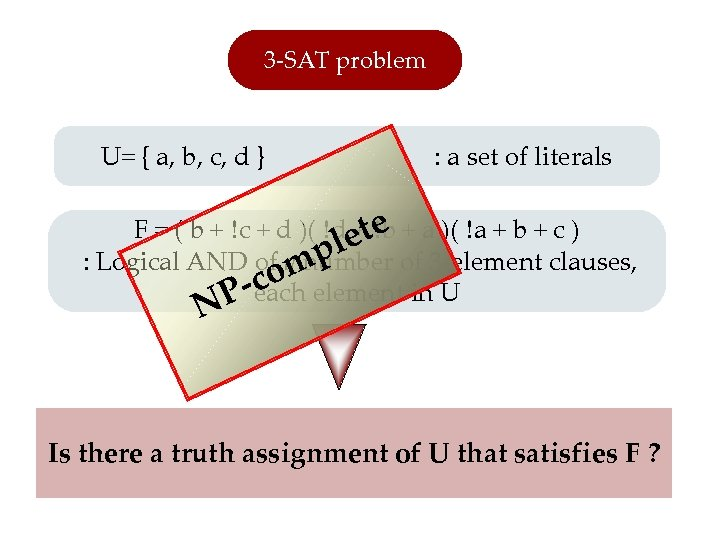3 -SAT problem U= { a, b, c, d } : a set of literals F = ( b + c + d )( d et b + a )( a + b + c ) +e pl of 3 -element clauses, : Logical AND of q number comelement in U P-each N Is there a truth assignment of U that satisfies F ?